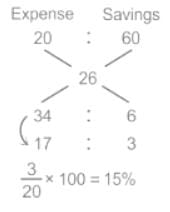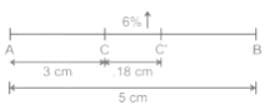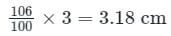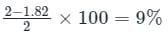SAT  >  Test: Percentages - 1

# Test: Percentages - 1

Test Description

## 10 Questions MCQ Test Mathematics for SAT | Test: Percentages - 1

Test: Percentages - 1 for SAT 2023 is part of Mathematics for SAT preparation. The Test: Percentages - 1 questions and answers have been prepared according to the SAT exam syllabus.The Test: Percentages - 1 MCQs are made for SAT 2023 Exam. Find important definitions, questions, notes, meanings, examples, exercises, MCQs and online tests for Test: Percentages - 1 below.
Solutions of Test: Percentages - 1 questions in English are available as part of our Mathematics for SAT for SAT & Test: Percentages - 1 solutions in Hindi for Mathematics for SAT course. Download more important topics, notes, lectures and mock test series for SAT Exam by signing up for free. Attempt Test: Percentages - 1 | 10 questions in 15 minutes | Mock test for SAT preparation | Free important questions MCQ to study Mathematics for SAT for SAT Exam | Download free PDF with solutions
 1 Crore+ students have signed up on EduRev. Have you?
Test: Percentages - 1 - Question 1

### The price of wheat is reduced by 4%. How many more or less kg of wheat can now be bought for the money which was sufficient to buy 48 kg wheat earlier?

Detailed Solution for Test: Percentages - 1 - Question 1

Given:

The price of wheat is reduced by 4%.

Assumption:

Let the price of wheat be Rs.100/kg.

Calculation:

The price of 48 kg wheat  = 4800

As price is reduce by 4% it means that it became 96% of initial 100% hence,

After price decrease = 4800/96 = 50 kg

Hence, the required quantity of wheat = (50 – 48) = 2 kg more.

Test: Percentages - 1 - Question 2

### The difference between the 30% of a number and 22% of that number is 4800. What is the 18% of that number?

Detailed Solution for Test: Percentages - 1 - Question 2

Given:

Difference between the 30% of a number and 22% of the same number = 4800

Calculation:

Let the number be P

According to the question,

30% of P – 22% of P = 4800

⇒ 8% of P = 4800

⇒ P = 60,000

And, 18% of P = (18/100) × 60,000 = 10,800

∴ 18% of P is 10,800

Test: Percentages - 1 - Question 3

### Kamal saves x% of her monthly income. When her monthly expenditure is increased by 20% and the monthly income is increased by 26%, then her monthly savings increased by 60%. What is the value of x?

Detailed Solution for Test: Percentages - 1 - Question 3

Given:

Kamal saves x% of her income

Increase in his income = 26%

Increase in his expenditure = 20%

Increase in saving = 60%

Concept used:

Income = Saving + Expenditure

Calculations:

Let income of Kamal be 100

Saving of Kamal = 100 × (x/100) = x

Expenditure of Kamal = 100 – x

Kamal's salary after increment = 100 × (126/100) = 126

Kamal's expenditure after increment = (100 – x) × 120/100 = (100 – x) × 6/5

New Saving = 126 – (100 – x) × 6/5      ----(1)

New saving after increment = x × 160/100

New saving after increment = 8x/5      ----(2)

From eq. (1) and eq. (2)

⇒ 126 – (100 – x) × 6/5 = 8x/5

⇒ 126 – 120 + 6x/5 = 8x/5

⇒ 6 = (8x/5) – (6x/5)

⇒ 6 = (8x – 6x)/5

⇒ 30 = 2x

⇒ x = 15

∴ The value of x is 15
Shortcut Trick:Test: Percentages - 1 - Question 4

A and B are two fixed points 5 cm apart and C is a point on AB such that AC is 3 cm. If the Length of AC is increased by 6%, the length of CB is decreased by how many %?

Detailed Solution for Test: Percentages - 1 - Question 4Increase in AC = 6%

Increase in AC =Decrease in CB = 5 - 3.18 = 1.82 cm

Decrease = 2 - 1.82 = 0.18 cm

So Percentage decreaseTest: Percentages - 1 - Question 5

In an election, there is neck to neck competition between two candidates A and B. Candidate A got 54% of valid votes and won by 3200 votes. If 20% of the votes cast in the election are invalid. Then find the total votes cast in the election.

Detailed Solution for Test: Percentages - 1 - Question 5

Given:

The difference of votes in Candidates A and B = 3200

Concept used:

By using the concept of percentage

Calculation:

Let the total number of valid votes cast in the election be x.

⇒ Votes got by A = 54/100 × x

⇒ Votes got by B = 46/100 × x

Required difference = Votes got by A – Votes got by B

⇒ 3200 = (54/100 × x) – (46/100 × x)

⇒ 3200 = 8/100 × x

⇒ x = 40000

⇒ 40000 = 80/100 × Total votes cast

The total votes cast in the election is 50000.

Test: Percentages - 1 - Question 6

800 g of sugar solution has 40% sugar in it. How much sugar should be added to make its proportion at 60% in the solution?

Detailed Solution for Test: Percentages - 1 - Question 6

Calculation:

Quantity of sugar in solution = 800 × (40/100) = 320 gm

Let the quantity of sugar added be x gm.

According to the question

⇒ (320 + x)/(800 + x) = 60/100

⇒ (320 + x)/(800 + x) = 3/5

⇒ (320 + x) × 5 = 3 × (800 + x)

⇒ 1600 + 5x = 2400 + 3x

⇒ 5x – 3x = 2400 – 1600

⇒ x = 400 gm
Shortcut Trick:

40%        100%

60%

40 : 20

2 : 1

2 unit = 800 g

1 unit = 400 g

Test: Percentages - 1 - Question 7

The difference between the 41% of a number and 33% of that number is 960. Then, what is the value of 33.33% of that number?

Detailed Solution for Test: Percentages - 1 - Question 7

Given:

The difference between the 41% of a number and 33% of that number is 960.

33.33% = 1 / 3

Calculation:

Suppose the number is N.

∴ 41% of N – 33% of N = 960

⇒ 8% of N = 960

⇒ N = (960 × 100) / 8

⇒ N = 12,000

According to question:

⇒ 33.33% of N = 1 / 3 of 12,000 = 4,000

Test: Percentages - 1 - Question 8

The price of sugar is increased by 25%. If a family wants to keep its expenses on sugar unaltered, then the familiy will have to reduce the consumption of sugar by:

Detailed Solution for Test: Percentages - 1 - Question 8

Given:

Increase % in the price of sugar = 25%

Formula used:

Expense = Price × Quantity

Calculation:

Let the initial expenses on sugar was Rs.100

Now, the price rises by 25%

⇒ New price = (100 + 100 × 25%) = 125

In order to keep the expense unaltered, Rs.25 has to be cut from Rs.125.

⇒ 125 - 25 = 100

∴ The decrease in the consumption = 25/125 × 100 = 20%
Alternate Method:

Reduction % = (Old quantity - New quantity)/Old quantity ×100

Let the price of 1 kg sugar = Rs.20

Let the the quantity of sugar bought = 5 kg

⇒ Expense = Price × Consumption = 20 × 5 = Rs.100

After increase of 25% in the price = Rs.20 × 125/100 = Rs.25

To keep the expense unchanged, that is Rs.100

⇒ New consumption = Rs.100/25 = 4 kg

Reduce in the consumption = 5 kg - 4 kg = 1 kg

∴ Percentage reduction in consumption = 1/5 × 100 = 20%
Shortcut Trick:

100 →  + 25% → 125 _____ - Y% → 100

Now, Y = 25/125 × 100 = 20%

Test: Percentages - 1 - Question 9

A student got 20% marks and failed by 72 marks. If he scores 40% marks then he gets 8 marks more than the passing marks. Find the passing marks.

Detailed Solution for Test: Percentages - 1 - Question 9

Given:

A student got 20% marks and failed by 72 marks. If he scores 40% marks then he gets 8 marks more than the passing marks.

Concept:

Percentage.

Solution:

Let total marks be x

20% of total marks + 72 = 40% of total marks - 8

⇒ (20/100)x + 72 = (40/100)x - 8

⇒ (x/5) + 72 = (2x/5) - 8

⇒ x/5 = 80

⇒ x = 400 = total marks

Hence, passing marks

⇒ (x/5) + 72 = (400/5) +72

⇒ 152

∴ The passing marks is 152.

Test: Percentages - 1 - Question 10

Two numbers are 50% and 75% lesser than a third number. By how much percent is the second number to be enhanced to make it equal to the first number?

Detailed Solution for Test: Percentages - 1 - Question 10

Let the third number = x

⇒ The first number = x - 50% of x = x/2

⇒ The second number = x - 75% of x = x/4

⇒ The percent by which second number has to be enhanced to make it equal to the first number = [(x/2 - x/4) / (x/4)] × 100 = 100%

(It can also be seen that x/4 is just half of x/2 so it has to be multiplied twice to become the second number which is 100%)

Alternate method

Let, 3rd number be 100

Hence, 2nd number will be 25 and 1st number will be 50

Now, 2nd number should be increased by 25 to make it equal to 1st number

∴ required percentage = 25/25 × 100 = 100%

## Mathematics for SAT

172 videos|94 docs|66 tests
 Use Code STAYHOME200 and get INR 200 additional OFF Use Coupon Code
Information about Test: Percentages - 1 Page
In this test you can find the Exam questions for Test: Percentages - 1 solved & explained in the simplest way possible. Besides giving Questions and answers for Test: Percentages - 1, EduRev gives you an ample number of Online tests for practice

## Mathematics for SAT

172 videos|94 docs|66 tests